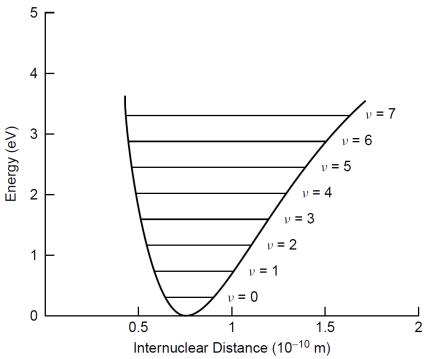Home Technology Molecular energy level

# Molecular energy level

Molecular energy levelMolecular energy level

The energy level structure formed by various motion states inside the molecule. The internal motion of the molecule includes electronic motion, molecular vibration and molecular rotation. Their energy is all quantized, so electronic energy levels, vibration energy levels and rotational energy levels can be formed. The electronic energy level of a molecule is on the order of 10 electron volts (eV), which is similar to the energy level of an atom; the vibrational energy level of a molecule is approximately 0.1 times the electronic energy level, and the rotational energy level of a molecule is approximately m/M of the electronic energy level Times, where m is the mass of electrons and M is the mass of typical molecules. Since the mass of a typical molecule is thousands to ten thousand times larger than the mass of an electron, the molecular vibrational energy level is 0.1 electron volt (eV) and the rotational energy level is 0.001 eV. Therefore, the energy level of a molecule is more complex than that of an atom. This determinant has a much richer spectrum than atoms.

This article is from the network, does not represent the position of this station. Please indicate the origin of reprint